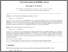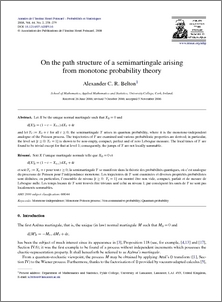# On the path structure of a semimartingale arising from monotone probability theory

Belton, Alexander C. R. (2008) On the path structure of a semimartingale arising from monotone probability theory. Annales de l'Institut Henri Poincaré (B) Probabilités et Statistiques, 44 (2). pp. 258-279. ISSN 0246-0203Preview
PDF
AIHP116.pdf - Published Version

Download (287kB)

## Abstract

Let X be the unique normal martingale such that X_0 = 0 and d[X]_t = (1 - t - X_{t-}) dX_t + dt and let Y_t := X_t + t for all t >= 0; the semimartingale Y arises in quantum probability, where it is the monotone-independent analogue of the Poisson process. The trajectories of Y are examined and various probabilistic properties are derived; in particular, the level set {t >= 0 : Y_t = 1} is shown to be non-empty, compact, perfect and of zero Lebesgue measure. The local times of Y are found to be trivial except for that at level 1; consequently, the jumps of Y are not locally summable.

Item Type: Journal Article Annales de l'Institut Henri Poincaré (B) Probabilités et Statistiques To appear in Annales de l'Institut Henri Poincare (B) Probability and Statistics; accepted in final form: 5th November 2006. Posted on the arXiv: 24th September 2007. RAE_import_type : Internet publication RAE_uoa_type : Pure Mathematics /dk/atira/pure/researchoutput/libraryofcongress/qa Faculty of Science and Technology > Mathematics and Statistics 2398 ep_importer 01 Apr 2008 08:43 Yes Published 22 Aug 2019 00:27 https://eprints.lancs.ac.uk/id/eprint/2398

### Actions (login required)View Item